# Mathematical integrals (Integration) in MT5 . ( How to do it)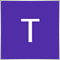310

i tried using ALGLIB but can't figure out how to accomplish this . The modified alglib does not have autogkintegrate() function and according to documentation it might have been replaced by autoGKIteration()

Since Alglib does not seem to be able to deal with Semi-Infinite intervals( a , +inf ) .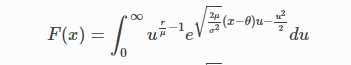So the way i thought to go about it is to have  integral  mapped and then integrated using autogksmooth()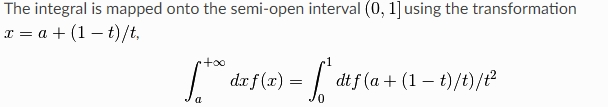Need help on how to integrate the following function in MT5 . I  looked at Alglib  integration.mqh but i could not figure out how to do it. Any help or pointers  will be great. I would like to use it in a test EA to perform a quick Quadrature integration. i looked at various quadpack C/C++ implementations  but they use templates and standard Full C++ , which won't really work in MT5

1. Can alglib be used ? if yes , how ? any sample code in MT5 ?

2. if Alglib can't be used then what would be the best way to go  about it ? any sample code in MT5  , please . Do i need to write an integration function from scratch ?  mql5 is not a full C++ language but a stripped specialized and customized C++ its so limited and that creates a nightmare since you can't really reuse any available standard C++ routines.

According to alglib samples , i have tried to test a toy sample but with no success.

```#include <Math/Alglib/alglib.mqh>
#include <Math/Alglib/integration.mqh>

CAutoGK myintegral;
CAutoGKState mystate;
CAutoGKReport myrep;

/*
Thus, the integration algorithm is used in accordance in the following order:

1. AutoGKState data structure preparation by means of one of the
algorithm initialization subrotuines (AutoGKSmooth, AutoGKSmoothW, AutoGKSingular).

2. AutoGKIteration subroutine call.

3. If False is returned from the subroutine, then the algorithm operation is completed
and integral is successfully calculated (the integral itself can be obtained
by calling the MinLMResults subprogram).

4. If True is returned from the subroutine, the latter will make a request for information on the function.
Calculate function value at State.X and save it in the State.F field. Then call AutoGKIteration again.
*/

void OnTick()
{

//
// This example demonstrates integration of f=exp(x) on [0,1]:
// * first, autogkstate is initialized
// * then we call integration function
// * and finally we obtain results with autogkresults() call
//
double lower_a = 0;
double upper_b = 1;

double my_v = 1; // this just a test

myintegral.AutoGKSmooth( lower_a, upper_b, mystate);

//autogkintegrate(mystate, int_function_1_func);  -----> Does not exist in Metatrader 5 version of Alglib
myintegral.AutoGKIteration(mystate);

myintegral.AutoGKResults(mystate, my_v, myrep);

Print("Interval = ", myrep.m_nintervals, "  nev = ", myrep.m_nfev);

}
//+------------------------------------------------------------------+

void int_function_1_func(double x, double xminusa, double bminusx, double &y)
{
// this callback calculates f(x)=exp(x)
y = exp(x);
}
```310

```#include <Math/Alglib/alglib.mqh>
#include <Math/Alglib/integration.mqh>
#include <Math/Alglib/ap.mqh>
#include <Math/Alglib/alglibinternal.mqh>
#include <Math/Alglib/linalg.mqh>
#include <Math/Alglib/specialfunctions.mqh>

CAutoGK myintegral;
CAutoGKState mystate;
CAutoGKReport myrep;

/*
Thus, the integration algorithm is used in accordance in the following order:

1. AutoGKState data structure preparation by means of one of the
algorithm initialization subrotuines (AutoGKSmooth, AutoGKSmoothW, AutoGKSingular).

2. AutoGKIteration subroutine call.

3. If False is returned from the subroutine, then the algorithm operation is completed
and integral is successfully calculated (the integral itself can be obtained
by calling the MinLMResults subprogram).

4. If True is returned from the subroutine, the latter will make a request for information on the function.
Calculate function value at State.X and save it in the State.F field. Then call AutoGKIteration again.
*/

// typedef a function
typedef void (*Tfunc)(double , double , double , double );

// prototype a function
void my_func(double , double , double , double );

void OnTick()
{

//
// This example demonstrates integration of f=exp(x) on [0,1]:
// * first, autogkstate is initialized
// * then we call integration function
// * and finally we obtain results with autogkresults() call
//

// declare a function pointer
Tfunc ptr_f;

// initialize the function pointer with a function address
ptr_f = my_func;

//Initialization of integral parameters:
//double x;
//double y;
double a = 0;
double b = 1;
double xminusa = 0.00001;
double bminusx = 0.00010;
double v;

mystate.m_f = ptr_f; //function
mystate.m_a = a;
mystate.m_b = b;
mystate.m_xminusa = xminusa;
mystate.m_bminusx = bminusx;

/*
//mystate.m_wrappermode = 1;
//mystate.m_x = x;
//mystate.m_a = a;
//mystate.m_b = b;
//mystate.m_alpha = alpha;
//mystate.m_beta = beta;
//mystate.m_xwidth= xwidth;
//mystate.m_internalstate
//mystate.m_needf
//mystate.m_nfev
//mystate.m_nintervals
//mystate.m_rstate
//mystate.m_terminationtype
//mystate.m_v

//--- allocation
//ArrayResizeAL(mystate.m_rstate.ra,11);
//mystate.m_rstate.stage=-1;
*/

myintegral.AutoGKSmooth( a, b, mystate);

myintegral.AutoGKIteration(mystate);

myintegral.AutoGKResults(mystate, v, myrep);

Print("Interval = ", myrep.m_nintervals, "  nev = ", myrep.m_nfev);

}

}
//+------------------------------------------------------------------+

void my_func(double x, double xminusa, double bminusx, double &y)
{
// this callback calculates f(x)=exp(x)
y = exp(x);
}
```

Tried this version of the code to integrate a simple function but that did not work . Get an error  "ptr_f" -type mismatch. Any Help please1673

`mystate.m_f = ptr_f; //function`

This tries to assign a function pointer to a double, which is illegal. If you need the function to be called via its pointer, use it like the function name itself, with parentheses.

```// prototype a function
void my_func(double , double , double , double );```
```void my_func(double x, double xminusa, double bminusx, double &y)
{
// this callback calculates f(x)=exp(x)
y = exp(x);
}```

Your function definition does not match your prototype declaration. In fact if you'd try to call that prototype you'd get a 'function must have a body' error.310

lippmaje:

This tries to assign a function pointer to a double, which is illegal. If you need the function to be called via its pointer, use it like the function name itself, with parentheses.

Your function definition does not match your prototype declaration. In fact if you'd try to call that prototype you'd get a 'function must have a body' error.

Thanks @lippmaje , how would you go about doing it , in this sample code ? . I tried to modify this sample code to the one below but still no integration being done

```#include <Math/Alglib/alglib.mqh>
#include <Math/Alglib/integration.mqh>
#include <Math/Alglib/ap.mqh>
#include <Math/Alglib/alglibinternal.mqh>
#include <Math/Alglib/linalg.mqh>
#include <Math/Alglib/specialfunctions.mqh>

CAutoGK myintegral;
CAutoGKState mystate;
CAutoGKReport myrep;

/*
Thus, the integration algorithm is used in accordance in the following order:

1. AutoGKState data structure preparation by means of one of the
algorithm initialization subrotuines (AutoGKSmooth, AutoGKSmoothW, AutoGKSingular).

2. AutoGKIteration subroutine call.

3. If False is returned from the subroutine, then the algorithm operation is completed
and integral is successfully calculated (the integral itself can be obtained
by calling the MinLMResults subprogram).

4. If True is returned from the subroutine, the latter will make a request for information on the function.
Calculate function value at State.X and save it in the State.F field. Then call AutoGKIteration again.
*/

// typedef a function
//typedef double (*Tfunc)(double , double , double , double );

// prototype a function
//double my_func(double , double , double , double );

void OnTick()
{

//
// This example demonstrates integration of f=exp(x) on [0,1]:
// * first, autogkstate is initialized
// * then we call integration function
// * and finally we obtain results with autogkresults() call
//

// declare a function pointer
//Tfunc ptr_f;

// initialize the function pointer with a function address
//ptr_f = my_func;

//Initialization of integral parameters:
double x;
double y;
double a = 0;
double b = 1;
double xminusa = 0.0;
double bminusx =  1e-7;
double v;

mystate.m_x = x;
mystate.m_a = a;
mystate.m_b = b;
mystate.m_xminusa = xminusa;
mystate.m_bminusx = bminusx;
mystate.m_f = my_func(x, xminusa, bminusx , y ); //function

/*
//mystate.m_wrappermode = 1;
//mystate.m_a = a;
//mystate.m_b = b;
//mystate.m_alpha = alpha;
//mystate.m_beta = beta;
//mystate.m_xwidth= xwidth;
//mystate.m_internalstate
//mystate.m_needf
//mystate.m_nfev
//mystate.m_nintervals
//mystate.m_rstate
//mystate.m_terminationtype
//mystate.m_v

//--- allocation
//ArrayResizeAL(mystate.m_rstate.ra,11);
//mystate.m_rstate.stage=-1;
*/

myintegral.AutoGKSmooth( a, b, mystate);

myintegral.AutoGKIteration(mystate);

myintegral.AutoGKResults(mystate, v, myrep);

Print(" v = ", v ,"  Interval = ", myrep.m_nintervals, "  nev = ", myrep.m_nfev);

}
//+------------------------------------------------------------------+

double my_func(double x, double xminusa, double bminusx, double y)
{
// this callback calculates f(x)=exp(x)
y = exp(x);
return(y);
}
```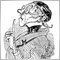Moderator
12275

Try to solve the equation like:

 ∫ 3x 2  d x   =   x 3+ c   .
Now one has to calculate x³ + c.1673

I have no experience with this lib.310

Carl Schreiber:

Try to solve the equation like:

 ∫ 3x 2  d x   =   x 3+ c   .
Now one has to calculate x³ + c.

@Carl, using your function example and the sample code above can you please illustrate if you can. i am having issues with integration . Can't seem to have it workingModerator
12275

My formula has nothing to do with your formula - it was meant only as an example how to 'eliminate' the integration sign and turn into a function that can easily be coded.

Otherwise you have to find a way to deal with dx and find a way to divide the area under the curve into strips that become narrower and narrower (limit value calculation) to add up their area.

BTW: if  alpha = 1 you can plot: 1+(1-x)/x and that looks pretty much like 1/x.

Try it here: https://rechneronline.de/funktionsgraphen/.

And:

 ∫ 1/x d x   =   ln|x| + c

from (https://en.wikipedia.org/wiki/Lists_of_integrals)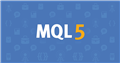Documentation on MQL5: Constants, Enumerations and Structures / Named Constants / Predefined Macro Substitutions
• www.mql5.com
//| Expert initialization function                                   | //| Expert deinitialization function                                 | //| Expert tick function                                             | //| test1                                                            |...310

Carl Schreiber:

My formula has nothing to do with your formula - it was meant only as an example how to 'eliminate' the integration sign and turn into a function that can easily be coded.

Otherwise you have to find a way to deal with dx and find a way to divide the area under the curve into strips that become narrower and narrower (limit value calculation) to add up their area.

BTW: if  alpha = 1 you can plot: 1+(1-x)/x and that looks pretty much like 1/x.

Try it here: https://rechneronline.de/funktionsgraphen/.

And:

 ∫ 1/x d x   =   ln|x| + c

from (https://en.wikipedia.org/wiki/Lists_of_integrals)

@ Carl, thanks for explaining310

When will MQL5 have  actual lambda functions  as in ( C++14 , C++17, or C++20 ) being  defined or declared using  "auto" keyword. I am really frustrated with Metaquotes in terms of mql5 language programming. its almost impossible to code a better simpler EA using simple mathematical calculations or simple C++14, C++17 or now C++20  standard guidelines. Most functions or structures allowed are very basic and sometimes does not lend itself to better coding of more simple EAs with better grounded mathematical , data analysis and statistical based codes

using typedef or others is sometimes a huge pain . it even becomes worse if you need to code your EA to sell on Metaquotes Market place you are required to code everything in a single file EA with no external dependencies ( which is fine if you have standard C++ implementation with common features and not stripped C++ version (specialized ) missing some basic C++14, C++17 or C++20 constructs )

if you try using ALGLIB then you face several issues which includes lack of complete support for the numerical library. There are no good samples to illustrate how to use ALGLIB with metaquotes because its a modified version of the Original ALGLIB  in order to work with Mql5 .

e.g such a sample using lambda functions.

```template<typename T>
void test_map_minf_pinf()
{
auto fun = [](T x) -> T { return x; };

using func_t = decltype(fun);

auto x_minf_pinf = [](auto t) -> T { return - T{1} / t + T{1} / (T{1} - t); };

for (int i = 0; i <= 100; ++i)
{
auto x = T{0.01} * i;
auto y = myNamespace::map_minf_pinf<T, func_t>(fun)(x);
Print(" x= ", x, " x_minf_pinf = ", x_minf_pinf(x), "  y = ",  y , "\n";
}
}```Documentation on MQL5: Constants, Enumerations and Structures / Named Constants / Predefined Macro Substitutions
• www.mql5.com
//| Expert initialization function                                   | //| Expert deinitialization function                                 | //| Expert tick function                                             | //| test1                                                            |...310

additional question: What version of standard C++ language is the current MQL 5 based on

1.   C++11

2.   C++14

3.   C++17

4.   C++20

5.   Objective-C++

Any idea ? I know that its a specialized , stripped  down and optimized version of some C++ language variant but which variant  ?  i have been trying to perform some simple integrations in MQL5 and its a complete mess .